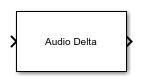# Audio Delta

Compute delta features

Since R2022b

•Libraries:
Audio Toolbox / Features

## Description

The Audio Delta block computes the delta of the input audio features. The delta is an approximation of the first derivative of the audio features with respect to time.

## Ports

### Input

expand all

Audio features, specified as a scalar, vector, matrix, or 3-D array. The delta computation operates along the first dimension. All other dimensions are treated as independent channels.

Data Types: `single` | `double`

### Output

expand all

Delta of audio features, returned as an array that is the same size and data type as the input.

Data Types: `single` | `double`

## Parameters

expand all

Window length over which to calculate delta, specified as an odd integer greater than 2.

• `Interpreted execution` –– Simulate model using the MATLAB® interpreter. This option shortens startup time but has a slower simulation speed than `Code generation`. In this mode, you can debug the source code of the block.

• `Code generation` –– Simulate model using generated C code. The first time you run a simulation, Simulink generates C code for the block. The C code is reused for subsequent simulations, as long as the model does not change. This option requires additional startup time, but the speed of the subsequent simulations is comparable to `Interpreted execution`.

## Block Characteristics

 Data Types `double` | `single` Direct Feedthrough `no` Multidimensional Signals `no` Variable-Size Signals `yes` Zero-Crossing Detection `no`

## Algorithms

The delta of an audio feature x is a least-squares approximation of the local slope of a region centered on sample x(k), which includes M samples before the current sample and M samples after the current sample.

`$delta=\frac{\sum _{k=-M}^{M}k\text{\hspace{0.17em}}x\left(k\right)}{\sum _{k=-M}^{M}{k}^{2}}$`

The delta window length defines the length of the region from M to M.